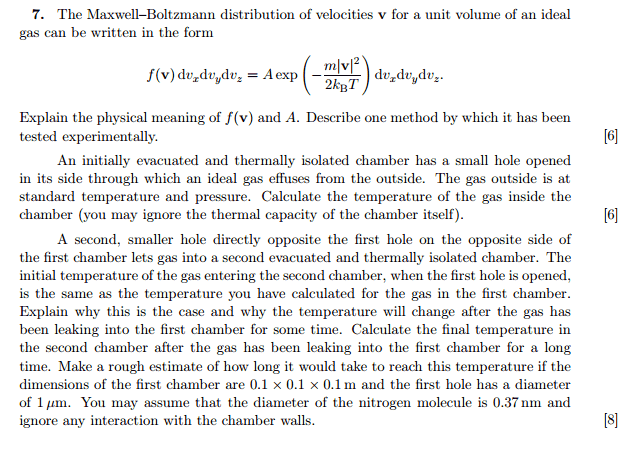# Effusion of gas between boxes

## Homework Statement

Part(a): explain the meaning of f(v) and A.

Part(b): Gas from surrounding air effuse into a box; find the temperature in the box.

Part (c): Explain why initial temperature of gas escaping from first box into second is the same as temperature in first box.

Part (d): Find equilibrium temperature of second box after a long time.## The Attempt at a Solution

Part(b)

$$Flux = \frac{1}{4}n<v> = \frac{1}{4} \frac {P_{air}}{kT_{air}} <v_{air}>$$

I assume RHS is constant, so flux entering the chamber is constant as the surrounding air is much more massive than the box.

$$\frac{PV}{kT} = \frac{1}{4} \frac {P_{air}}{kT_{air}} <v_{air}>$$

If the box is thermally isolated, I can't assume temperature of box = temperature of air. Also, I do not know the pressure in the box. Without the pressure, I can't solve for temperature.

Part(c)

I suppose temperature is the measure of average KE of molecules, so the first few molecules escaping into the second box should have the same temperature as molecules in the first box. After some time, the temperature changes because pressure inside second box changes?

Part(d)

After a long time, equilibrium has been established.

Flux out of second box = flux into second box
$$\frac{1}{4}n_2<v_2> = \frac{1}{4}n_1<v_1>$$
$$\frac {P_2}{T_2} (\sqrt{T_2}) = \frac {P_1}{T_1} (\sqrt{T_1})$$
$$\frac {P_2}{\sqrt{T_2}} = \frac {P_1}{\sqrt{T_1}}$$

I'm not sure what the pressures are...

I've been thinking, is it okay to assume for part (b) that the pressure in both boxes are equal?

TSny
Homework Helper
Gold Member
If the box is thermally isolated, I can't assume temperature of box = temperature of air. Also, I do not know the pressure in the box. Without the pressure, I can't solve for temperature.

The temperature of an ideal gas is related to the average kinetic energy per molecule. Try to determine the average energy per molecule of the molecules that pass through the hole into chamber 1. This can be done using the Maxwell-Boltzmann distribution ##f(\vec{v}) \equiv f(v_x, v_y, v_z)##.

(The average energy per molecule passing through the hole is not the same as the average energy per molecule in the air outside.)

The temperature of an ideal gas is related to the average kinetic energy per molecule. Try to determine the average energy per molecule of the molecules that pass through the hole into chamber 1. This can be done using the Maxwell-Boltzmann distribution ##f(\vec{v}) \equiv f(v_x, v_y, v_z)##.

(The average energy per molecule passing through the hole is not the same as the average energy per molecule in the air outside.)

The resulting speed distribution of particles hitting the wall is non-maxwellian.
In fact, it incurs an additional factor of v, so speed distribution ##f_{(v^2)} \propto v^3 e^{-\alpha v^2} = Av^3 e^{-\alpha v^2}##.

To find coefficient A, i integrate from 0 to infinity and set = 1.

$$\frac{1}{2}m<v^2> = \frac{1}{2}m \int_0^{\infty}A v^5e^{-\alpha v^2} dv$$

$$<E'> = \frac{1}{2}m \int_0^{\infty}A v^5e^{-\alpha v^2} dv = 2kT$$

To recast this in the form of ##<E'> = \frac{3}{2}kT'##:

$$2kT = \frac{3}{2}kT'$$

Thus, ##T'= \frac{4}{3}T##

Last edited:
TSny
Homework Helper
Gold Member
EDITED. The answer looks correct to me for monatomic molecules, but I'm not following your calculation. How did you take into account that you only want the average energy per molecule that passes through the hole? I guess your starting expression ##Av^3 e^{-\alpha v^2}## comes from a separate analysis for the rate at which molecules would pass through the hole.

There is an additional complication if you want to treat the molecules as diatomic with rotational degrees of freedom. (The problem statement mentions nitrogen.) I don't know if you are meant to take that into account.

Last edited: